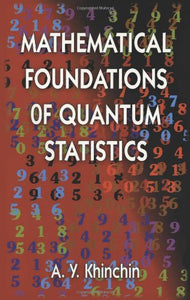# Mathematical Foundations Of Quantum Statistics (Dover Books On Mathematics)

• Publish Date: 2011-11-02
• Binding: Paperback
• Author: A. Y. Khinchin;Mathematics
Regular price \$70.81 Sale price \$33.26

Attention: For textbook, access codes and supplements are not guaranteed with used items.

In this high-level mathematical treatment, a distinguished Russian mathematician introduces the mathematical underpinnings of quantum statistics in a manner accessible to both mathematicians and physicists. The book is aimed primarily at mathematicians, however, who will need only an elementary grasp of quantum physics to appreciate the author's well-organized and clearly written presentation. Beginning with preliminary concepts in the theory of probability, the text opens with a discussion and complete proofs of the limit theorems used in the main sections of the book. Proceeding to preliminary concepts and general principles of quantum mechanics, it examines the basis of the computational methods of quantum statistics and illustrates the means to achieve the statisticians' most important initial task: the determination of the mean values of occupation numbers. In addition, the foundations of the statistics of photons and material particles receive ample review.
Supplements to the concluding chapter on thermodynamics explore the distribution of a component and its energy, the principle of canonical averaging, symmetric functions on multidimensional surfaces, and other related topics.